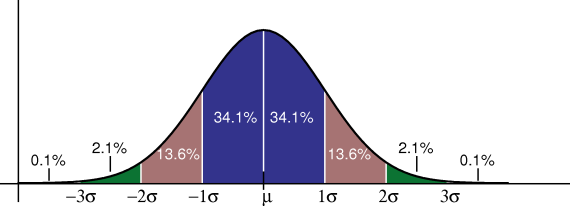# Appendix C: Gaussian Distribution

## Gaussian distribution.

The Gaussian (normal) distribution was historically called the law of errors. It was used by Gauss to model errors in astronomical observations, which is why it is usually referred to as the Gaussian distribution.

The probability density function for the standard Gaussian distribution (mean 0 and standard deviation 1) and the Gaussian distribution with mean μ and standard deviation σ is given by the following formulas.The cumulative distribution function for the standard Gaussian distribution and the Gaussian distribution with mean μ and standard deviation σ is given by the following formulas.As the figure above illustrates, 68% of the values lie within 1 standard deviation of the mean; 95% lie within 2 standard deviations; and 99.7% lie within 3 standard deviations.

## Central limit theorem.

Under generous technical conditions, the distribution of the sum of a large number of independent random variables (approximately) has a normal distribution.

In science and engineering, it is often reasonable to treat the error of an observation as the result of many small, independent, errors. This enables us to apply the central limit theorem and treat the errors as normal.

Caveat: central limit theorem typically only applies when close to the peak; may not apply in the tails.

The French physicist Gabriel Lippman wrote the following in a letter to Henri Poincare.

Tout le monde y croit cependent, car les experimenteurs s'imaginent que c'est un theorem de mathematiques, et les mathematiciens que c'est un fait experimental.

Everybody believes in the exponential law of errors: the experimenters, because they think it can be proved by mathematics; and the mathematicians, because they believe it has been established by observation.

## Poisson distribution.

A dam has a lifespan of 50 years. What is the chance that a 100-year flood will occur during once during the life of the dam? (Model a 100-year flood as occurring with probability 1/100 per year, or 1/36500 per day, or in the limit using a Poisson distribution).

1/2 exp(-1/2) = 30.33%.